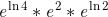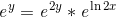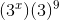# Exponentials, Logarithms & the Natural Log

Instructions:

Choose an answer and hit 'next'. You will receive your score and answers at the end.

question 1 of 3

### Simplify the following expression.Create Your Account To Take This Quiz

As a member, you'll also get unlimited access to over 75,000 lessons in math, English, science, history, and more. Plus, get practice tests, quizzes, and personalized coaching to help you succeed.

Try it risk-free for 30 days. Cancel anytime.

### 1. Solve the following equation for y = f(x).### 2. Simplify:Create your account to access this entire worksheet
A Premium account gives you access to all lesson, practice exams, quizzes & worksheets
Quizzes, practice exams & worksheets
Certificate of Completion
Create an account to get started

Test your ability to solve and simplify problems with exponentials, logarithms and natural log in this quiz and worksheet combo.

## Quiz & Worksheet Goals

In these practice assessments you'll be tested over your ability to:

• Solve equations with logarithms
• Simplify exponential expressions with natural log
• Find the age of a carbon sample using its half-life

## Skills Practiced

This quiz and worksheet allow students to test the following skills:

• Reading comprehension - ensure that you draw the most important information from the related calculus lesson
• Problem solving - use acquired knowledge of exponentials and logarithms to solve practice problems
• Knowledge application - use your knowledge of natural log, inverse functions and half-life to find the age of a substance

To learn more about how to use exponents and logs, you can check out the accompanying lesson Exponentials, Logarithms & the Natural Log. This lesson covers the following mathematical topics:

• Graphing exponentials
• Properties of exponentials
• Inverse functions
• Solving equations using natural log
Final ExamMath 104: Calculus
Status: Not Started
Chapter ExamGraphing and Functions
Status: Not Started

Support